## Microsoft word - new microsoft word document.doc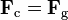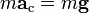Chapter – 7
Geostationary Satellite
A geostationary orbit (GEO) is a geosynchronous orbit directly above the Earth's equator (0° latitude), with a period equal to the Earth's rotational period and an orbital eccentricity of approximately zero. From locations on the surface of the Earth, geostationary objects appear motionless in the sky, making the GEO an orbit of great interest to operators of communications and weather satellites. Due to the constant 0° latitude and circularity of geostationary orbits, satellites in GEO differ in location by longitude only. Geostationary orbits are useful because they cause a satellite to appear stationary with respect to a fixed point on the rotating Earth. As a result, an antenna can point in a fixed direction and maintain a link with the satellite. The satellite orbits in the direction of the Earth's rotation, at an altitude of 35,786 km (22,236 mi) above ground. This altitude is significant because it produces an orbital period equal to the Earth's period of rotation, known as the sidereal day. Geostationary alttitude
In any circular orbit, the centripetal acceleration required to maintain the orbit is provided by the gravitational force on the satellite. To calculate the geostationary orbit altitude, one begins with this equivalence, and uses the fact that the orbital period is one sidereal day. By Newton's second law of motion, we can replace the forces F with the mass m of the object multiplied by the acceleration felt by the object due to that force: We note that the mass of the satellite m appears on both sides — geostationary orbit is independent of the mass of the satellite.So calculating the altitude simplifies into calculating the point where the magnitudes of the centripetal acceleration required for orbital motion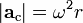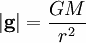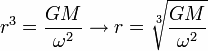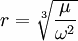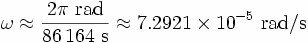and the gravitational acceleration provided by Earth's gravity are equal. The centripetal acceleration's magnitude is: where ω is the angular speed, and r is the orbital radius as measured from the Earth's center of mass. The magnitude of the gravitational acceleration is: where M is the mass of Earth, 5.9736 × 1024 kg, and G is the gravitational constant, 6.67428 ± 0.00067 × 10−11 m3 kg−1 s−2. The product GM is known with much greater accuracy than either factor; it is known as the geocentric gravitational constant µ = 398,600.4418 ± 0.0008 km3 s−2: The angular speed ω is found by dividing the angle travelled in one revolution (360° = 2π rad) by the orbital period (the time it takes to make one full revolution: one sidereal day, or 86,164.09054 seconds). This gives: The resulting orbital radius is 42,164 kilometres (26,199 mi). Subtracting the Earth's equatorial radius, 6,378 kilometres (3,963 mi), gives the altitude of 35,786 kilometres (22,236 mi).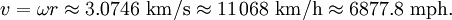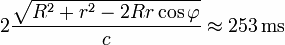Orbital speed (how fast the satellite is moving through space) is calculated by multiplying the angular speed by the orbital radius: Communications
Satellites in geostationary orbits are far enough away from Earth that communication latency becomes very high — about a quarter of a second for a one-way trip from a ground based transmitter to a geostationary satellite and then on to a ground based receiver, and close to half a second for round-trip communication between two earth stations. For example, for ground stations at latitudes of φ=±45° on the same meridian as the satellite, the one-way delay can be computed by using the cosine rule, given the above derived geostationary orbital radius r, the Earth's radius R and the speed of light c, as. This presents problems for latency-sensitive applications such as voice communication or online gaming.

Source: http://www.mudlle.ac.in/document/moodle/dt/chapter7.pdf

### Untitled

PPTP y L2TP por Marisabel Rodriguez Bilardo Los protocolos PPTP y L2TP nacieron para crear VPNs. Además de permitir crear túneles a través de Internet, pueden encriptar los datos enviados y autenticar a los usuarios. Las VPNs conectan sitios remotos en forma segura y ademásbajan costos porque utilizan redes públicas en vez de líneasPPTP y L2TP son idénticos en la c

### Microsoft word - liste over nominerede 2010_final.docx

DANISH BEAUTY AWARD 2010 De nominerede er… ÅRETS HÅRPRODUKT - LUKSUS/POPULÆR Aussie - 3 Minute Miracle Reconstructor Stuhr - Mild Family Shampoo Normalt/Tørt hår Syoss - Shine Boost Instant-Shine Treatment TRESemmé - Healthy Volume Shampoo Unique Products - Therapeutic Protein Shampoo ÅRETS HÅRPRODUKT - SALON/KLINIK/SPA Matrix - Biolage Colorcarethérapie Delicate Care Miljøcertifice